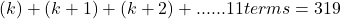The Graduate Record Examination, GRE, is a standardized test required for admission to graduate schools in the United States, Australia, Canada, and many other countries. It tests a variety of skills of students.

The Graduate Record Exam includes math questions to test your analytical skills. One type of question they ask is on a sequence of numbers.

The question below represents the type of question on sequence of numbers you can expect to see on the Graduate Record Examination (GRE):

Question: If the sum of 11 consecutive odd integers is 319. Find the 4th number.

Approach: First, we need to figure out how to generalize this into an equation. Let’s try to write like?

Well, this won’t really work because of 2 reasons:

a. k is any integer. So it can be even as well – which is not allowed

b. These are consecutive integers. But not consecutive ODD integers.

So, we need to find a way to represent an odd number. How about 2k+1? “2k” will always be even and when we add “1” to it, it will become odd. So 2k+1 is always odd.

Now, the next number to an odd number is an even number. But the next to next will be another (consecutive) odd. So, 2k+1+2=2k+3 is a consecutive odd number. Likewise, we can make a series as shown in the video.

All we have to do now is use the formula of the sum of Arithmetic progression and solve for k.